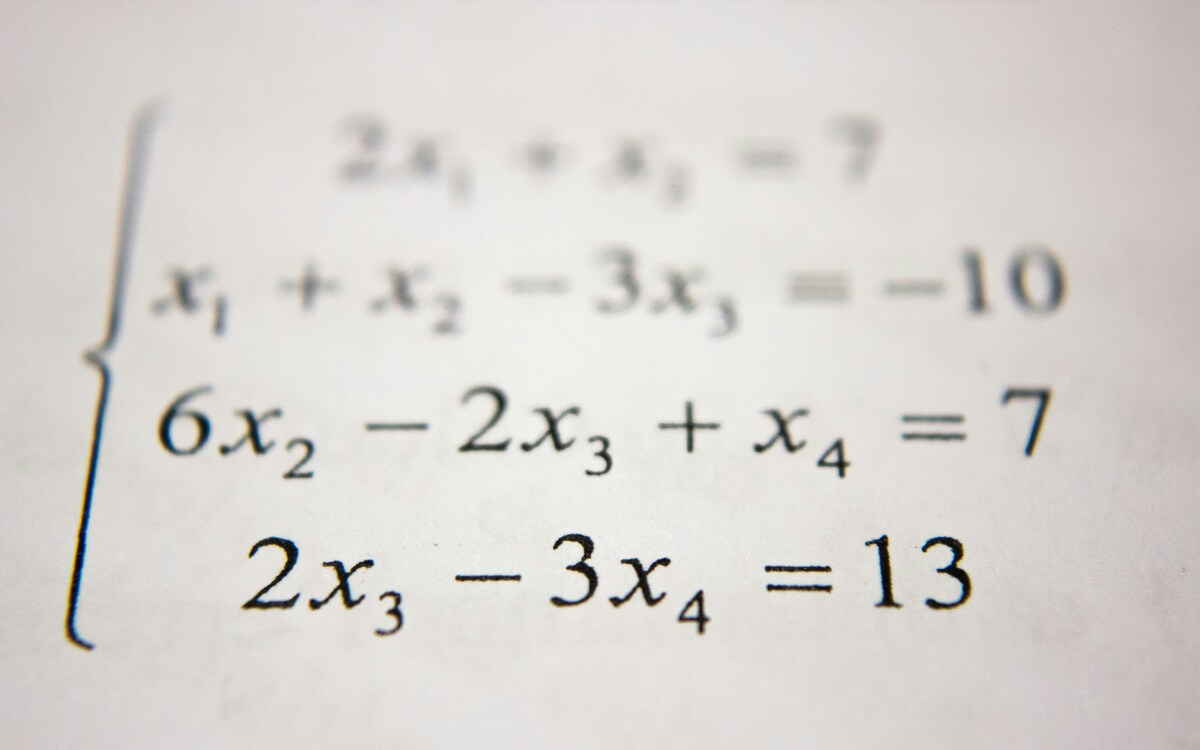# Fraction to Decimal Calculator

Fraction to Decimal Calculator: The Fraction to Decimal Converter over here is an online tool that gives the equivalent decimal value for a given fraction value. This handy tool allows users to provide input in the form of a rational number m/n where m, n are integers and n is not equal to zero and gives the decimal output separated by the period(.)

Here are some samples of fractions to decimal conversion calculations.

Related Calculators:

This online tool makes all your calculations faster and displays the conversion in a fraction of seconds. Apart from this, you can see solved examples showing the work step by step. Learn different ways to convert fraction to decimal in the coming modules like Long Division, Simplifying Methods, etc.

## What is meant by Fraction to Decimal?

In Mathematics, Fraction is defined as the ratio of two numbers. It means the ratio of numerator value to the denominator value. A decimal number is a number whose whole number and decimal part are separated by a decimal point. In order to change a fraction to decimal, simplify the fraction as much as possible. When the fraction is simplified we get the decimal number.

### Procedure to find Fraction to Decimal

You might need to convert a number to different forms to represent a number differently. There are several methods to convert a fraction to decimal. Check out some of the methods outlined below.

Long Division Method

Long Division provides a way to convert fraction to decimal form. You should identify the dividend and divisor first and then use those values in the long division. In the given fraction numerator will be the dividend and denominator will be the divisor. Represent the dividend and divisor in the form of long division. You likely need to add a decimal and zeros if the dividend is smaller than the divisor.

Solve the long division to convert from fractions to decimals.Therefore fraction 3/4 in decimal value is 0.75

Simplifying

An alternate method to convert fractions to decimals is to simplify the fraction. This needs a few steps. Initially, multiply the denominator to get to 100. before doing this step, try dividing 100 with the denominator to know the multiple and multiply both numerator and denominator by the multiple.

The last step is to move the decimal place on the new numerator to the left two places to change a fraction to its decimal value.

Example

Convert 1/4 to a decimal using the simplifying method?

Solution:

Firstly find the multiple to multiply the denominator to obtain 100

100 = 4*25

Multiple is 25. Multiply both the numerator and denominator by multiple and the result is as follows

1/4 = 25/100

Finally, move the numerator's decimal place to left two places to get the decimal value.

25.0 -> 0.25

Decimal Value of 1/4 is 0.25

### How to use Fraction to Decimal Calculator?

Procedure to use Fraction to Decimal Calculator is along the lines

• Firstly, enter the fraction value in the input field of the calculator.
• Now, click the enter button right after the input field or press enter in your keyboard.
• Finally, you will get the decimal value displayed on the screen in a fraction of seconds.### FAQs on Fraction to Decimal

1. What are the ways to convert fractions to decimals?

You can convert fractions to decimals using any of the methods like Long Division, Simplifying.

2. Where can I get Worked Examples on Fraction to Decimal Conversion?

You can get Worked Examples on Fraction to Decimal on our page.

3. How to Convert Fractions to Decimals easily?

Make use of our fraction to decimal calculator tool and get the output equivalent in decimal value for the given fraction value.

4. What is fraction 1/5 in decimal value?

In the given fraction numerator 1 is the dividend and denominator 5 is the divisor. As the dividend is smaller than the divisor place a decimal point and zeros. On doing the division we get the result as follows

1/5 = 0.2

Therefore fraction 1/5 in decimal value is 0.2